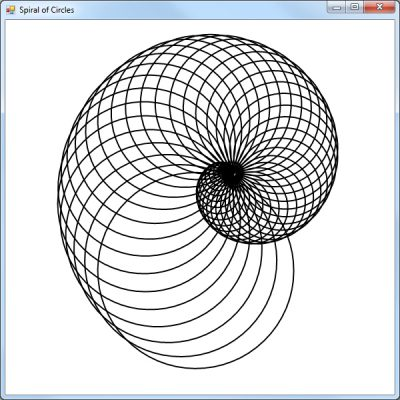# Turtle Graphics Spiral In C#

As another example of what you can do with graphics in C#, I decided to recreate a famous Turtle Graphics spiral design. I can’t find an official name for this classic design. I decided to call it Spiral of Circles. It has a nautilus shape but you won’t find any sample code by doing a search on “Turtle Graphics nautilus”. There is a version of this design using squares. I did have the code for creating this design using Python or Processing. It took a little experimentation to reproduce it in C#. Part of the solution was to realize that radians needed to be converted to degrees which is why I included the conversion functions in the code. This design requires a rotate and a translate transformation within a loop. I’m not sure you really need to use a GraphicsPath object because I tried that before I solved the other problems.

``````
using System;
using System.Collections.Generic;
using System.ComponentModel;
using System.Data;
using System.Drawing;
using System.Drawing.Drawing2D;
using System.Linq;
using System.Text;
using System.Windows.Forms;

namespace SpiralOfCircles
{
public partial class Form1 : Form
{

System.Drawing.Graphics graphicsObj;

public Form1()
{
InitializeComponent();
}

private void Form1_Paint(object sender, PaintEventArgs e)
{
graphicsObj = this.CreateGraphics();

// Set the SmoothingMode property to smooth the lines.
graphicsObj.SmoothingMode = System.Drawing.Drawing2D.SmoothingMode.AntiAlias;

// This centers what is drawn. Adjusted by 50 pixels.
graphicsObj.TranslateTransform((graphicsObj.VisibleClipBounds.Width / 2) + 50, (graphicsObj.VisibleClipBounds.Height / 2) - 50, MatrixOrder.Append);
// This scales everything up
graphicsObj.ScaleTransform(2, 2);

// Create pen.
Pen blackPen = new Pen(Color.Black, 1);

for (int i = 0; i < 50; i++)
{
graphicsObj.RotateTransform((float)degrees((-Math.PI / 18)));

// Create a GraphicsPath object
GraphicsPath path = new GraphicsPath();

// Draw a circle
path.AddEllipse(0, 0, -i * 3, -i * 3);

// Create a Matrix object
Matrix X = new Matrix();
X.Translate(i, 0);

// Apply transformation
path.Transform(X);
graphicsObj.DrawPath(blackPen, path);
}
}

/// <summary>
/// Function to convert degrees to radians
/// </summary>
/// <param name="degrees">degree value</param>
{
double radians = (Math.PI / 180) * degrees;
}

/// <summary>
/// Function to convert radians to degree
/// </summary>
/// <returns>equivalent degree value</returns>